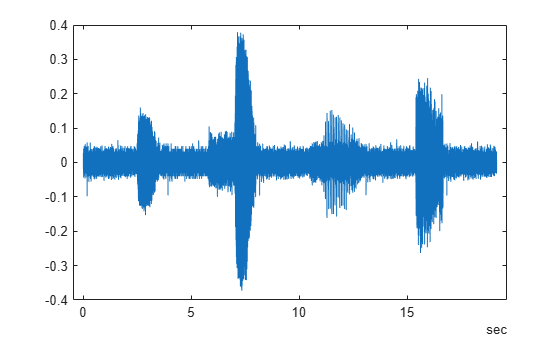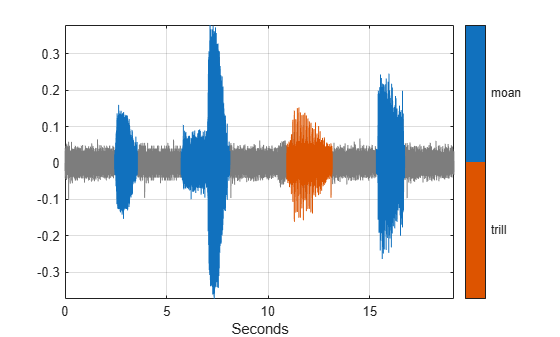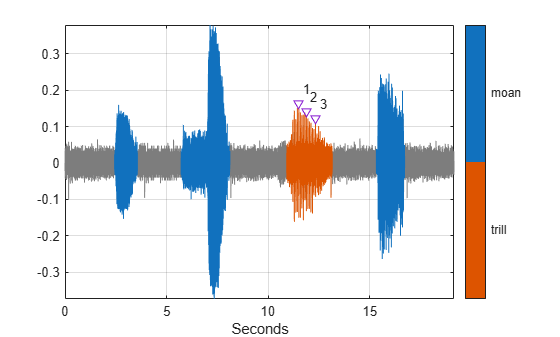Main Content

# Examine Labeled Signal Set

Load into the MATLAB® Workspace the MAT-file you created in the Label Signal Attributes, Regions of Interest, and Points example. Verify that the labeled signal set contains the definitions that you added using Signal Labeler.

```load Whale_Songs labelDefinitionsSummary(whalesongs)```
```ans=3×9 table LabelName LabelType LabelDataType Categories ValidationFunction DefaultValue Sublabels Tag Description ______________ ___________ _____________ ____________ __________________ ____________ ___________________________ ___ ____________________________ "WhaleType" "attribute" "categorical" {3x1 string} {["N/A" ]} {0x0 double} {0x0 double } "" "Whale type" "MoanRegions" "roi" "logical" {["N/A" ]} {0x0 double} {0x0 double} {0x0 double } "" "Regions where moans occur" "TrillRegions" "roi" "logical" {["N/A" ]} {0x0 double} {0x0 double} {1x1 signalLabelDefinition} "" "Regions where trills occur" ```

Verify that `TrillPeaks` is a sublabel of `TrillRegions`.

`labelDefinitionsHierarchy(whalesongs)`
```ans = 'WhaleType Sublabels: [] MoanRegions Sublabels: [] TrillRegions Sublabels: TrillPeaks ' ```

Retrieve the second member of the set. Retrieve the names of the timetable variables.

```song = getSignal(whalesongs,2); summary(song)```
```RowTimes: Time: 76579x1 duration Values: Min 0 sec Median 9.5722 sec Max 19.144 sec TimeStep 0.00025 sec Variables: whale2: 76579x1 double Values: Min -0.37326 Median 0 Max 0.37914 ```

Plot the signal.

```t = song.Time; sng = song.whale2; plot(t,sng)```### Visualize Labeled Regions

Use a `signalMask` object to display and identify the regions of interest that you labeled. For better display, change the label values from logical to categorical.

```mvals = getLabelValues(whalesongs,2,'MoanRegions'); mvals.Value = categorical(repmat("moan",size(mvals,1),1)); tvals = getLabelValues(whalesongs,2,'TrillRegions'); tvals.Value = categorical(repmat("trill",size(tvals,1),1)); msk = signalMask([mvals;tvals],'SampleRate',1/seconds(t(2)-t(1))); plotsigroi(msk,sng)```### Visualize Labeled Points

Display and identify the trill peaks that you labeled.

```pk = getLabelValues(whalesongs,2,{'TrillRegions','TrillPeaks'}); locs = zeros(size(pk,1),1); for kj = 1:length(locs) locs(kj) = find(seconds(t) == pk.Location(kj)); end hold on plot(seconds(t(locs)),sng(locs)+0.01,'v') text(seconds(t(locs))+0.2,sng(locs)+0.05,int2str(cell2mat(pk.Value))) hold off```## Support

#### Deep Learning for Signal Processing with MATLAB

Download white paper